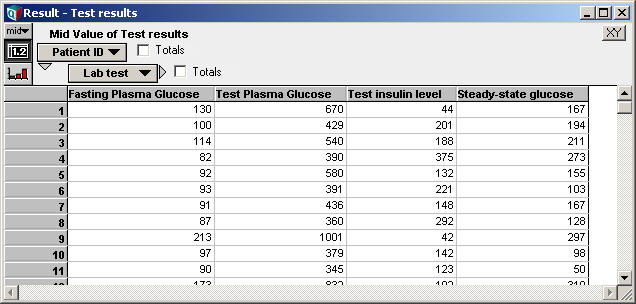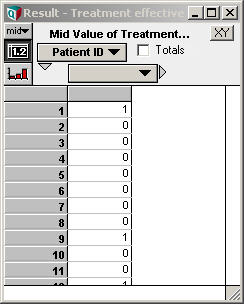# Probit Regression

Requires Analytica Optimizer

A probit model relates a continuous vector of dependent measurements to the probability of a binomial (i.e. 0, 1-valued) outcome. In econometrics, this model is sometimes called the Harvard model. The Probit_Regression function infers the coefficients of the model from a data set, where each point in the training set is classified as 0 or 1.

Probit regression is very similar to Logistic Regression. Both are used to fit a binomial outcome based on a vector of continuous dependent quantities. They differ in their use of the link function. For the relationship, see these Wikipedia articles on the Wikipedia Generalized Linear Model and the probit model.

## Probit_regression(Y, B, I, K)

Given a set of data points, indexed by «I», with each point classified as 0,1 in the «Y» parameter, and a set of basis terms, «B», containing the dependent variables (where the vector of dependent variables is indexed by «K»), the Probit_Regression function finds and returns the set of coefficients for the probit model:

$\displaystyle{ Pr(Y=1|B=b) = \Theta^{-1}\left(\sum_k c_k b_k\right) }$

where $\displaystyle{ \Theta^{-1} }$ is the inverse cumulative normal distribution function.

The basis, «B», is a function of the dependent variables in your data. Each element along «K» of the basis vector may be an arbitrary, even non-linear, combination of the data in your data set. However, the number of terms in the basis should be kept small relative to the number of data point in your data set.

## Library

Generalized Regression (Generalized Regression.ana)

## Example

Suppose you want to predict the probability that a particular treatment for diabetes is effective given several lab test results. Data is collected for patients who have undergone the treatment, as follows, where the variable Test_results contains lab test data and Treatment_effective is set to 0 or 1 depending on whether the treatment was effective or not for that patient:Using the data directly as the regression basis, the logistic regression coefficients are computed using:

Variable c := Probit_Regression(Treatment_effective, Test_results, Patient_ID, Lab_test)

We can obtain the predicted probability for each patient in this testing set using:

Variable Prob_Effective := CumNormal(Sum(c*Test_results, Lab_Test))

If we have lab tests for a new patient, say New_Patient_Tests, in the form of a vector indexed by Lab_Test, we can predict the probability that treatment will be effective using:

CumNormal(Sum(c*New_patient_tests, Lab_test))

See the example for Logistic_Regression for a further elaboration of this example, with additional notes on potential over-fitting problems that are common with this type of data analysis.

## History

In Analytica 4.5, this function has been superseded by the ProbitRegression function.